# A Sine Wave

 Tip An ANN can be used to eliminate noise from a sine wave or other kind of waveform. For these problems, the training set must include sample points of the wave. Additionally, each training case may include the same wave at a different phase. The results of this section have the intention to illustrate the ANN capacity to eliminate noise, even though there are other techniques using digital signal processing that can also be used.

 Tip One easy way to contaminate a signal is by adding white noise of similar amplitude. For instance to contaminate z = sin(x) with noise, the noise amplitude must be from -1 to 1. Thus, y = 0.8*sin(x) + 0.2*noise creates the new signal y with 20% of noise.

 Problem 1 Create a New Project SineWave to build an appropriate training set for learning of the sine wave z = sin(x) using a ANN for auto-association as shown in the figure. Use 2048 training cases and 64 inputs.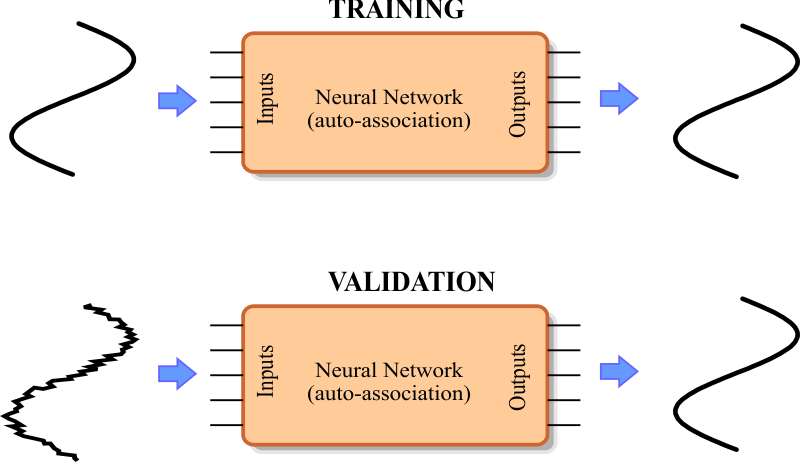Solution 1 After editing the file,Run click the button to execute the code. If you do not have any errors, the training set will be generated and displayed on the variable list and the file list. Click on the file to see its contents. Use the scroll bar at the right to visualize each training case. Each case should be a sine wave at a different phase. Observe that in this specific case the training set input and target are the same.

 SineWave\BuildTrainSet.lab int numCases = 2048; int numInputs = 64; double range = 2.0*3.1415926; double deltaInput = range/numInputs; double deltaCase = range/numCases; Matrix trainSet; trainSet.Create(numCases, numInputs); int i; int j; double phase = 0.0; for(i=0; i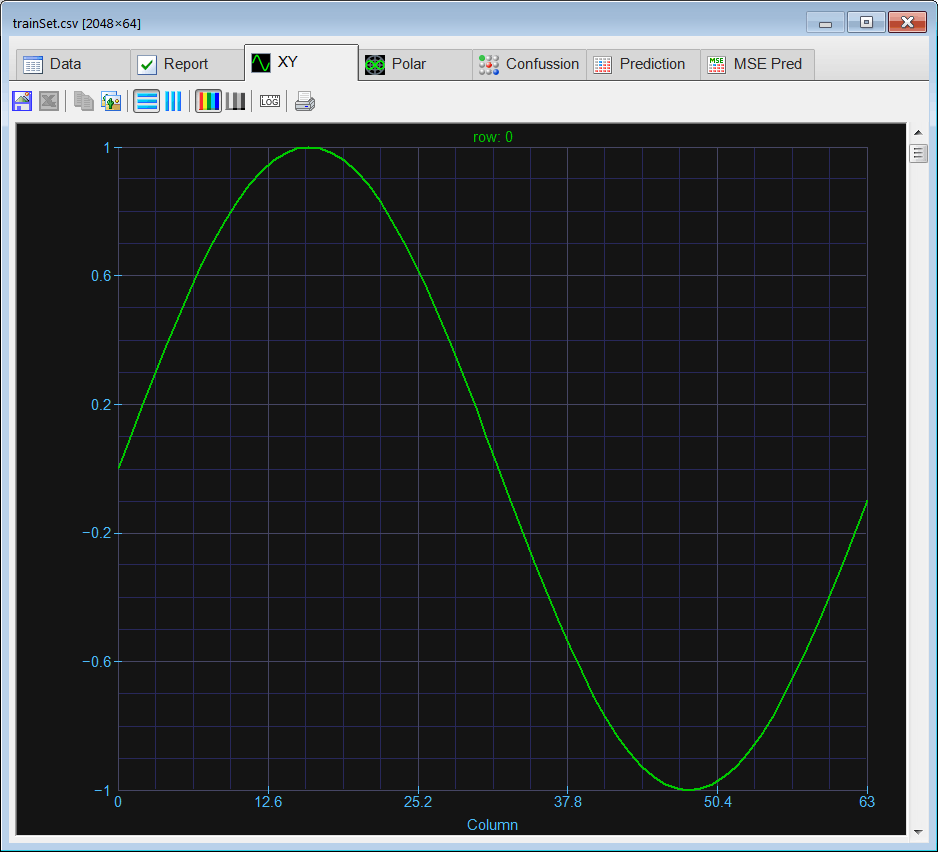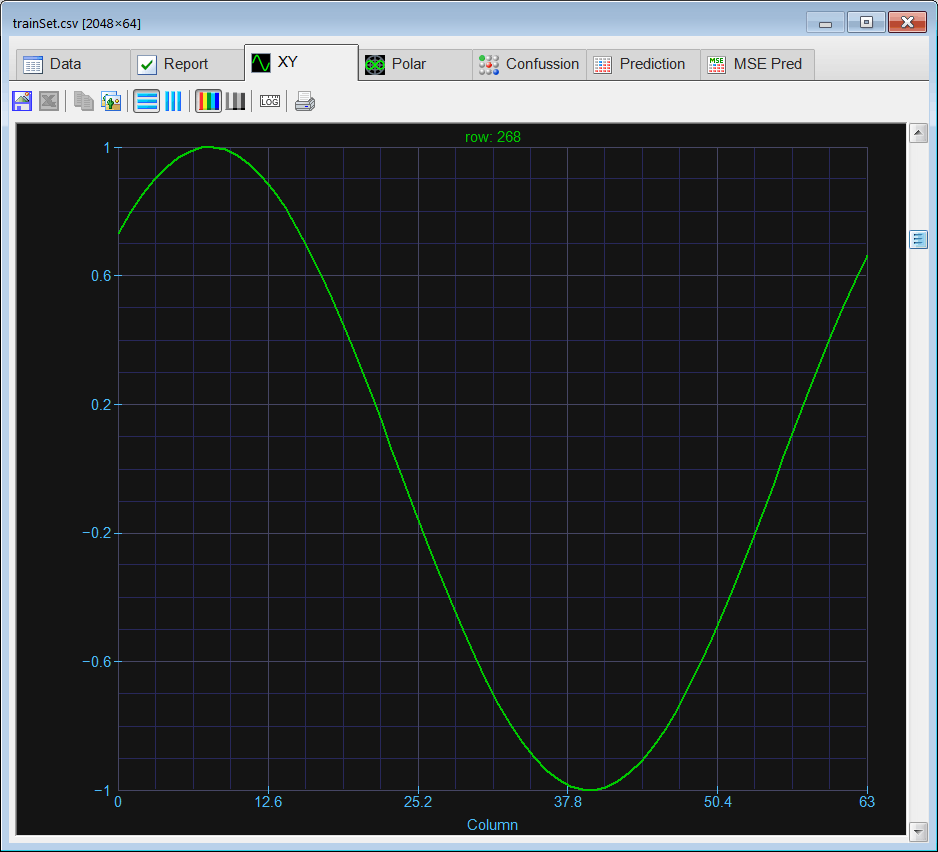Problem 2 Edit the BuildValidSet.lab file to build the validation set using the training set. Contaminate the training set with 10% of noise to create the validation set.

 Solution 2 After editing the code,Run click the button to execute the code. If you do not have any errors, the validation set will be generated and displayed on the variable list and the file list. Use the scroll bar at the right to visualize each validation case. Each case should be a noisy sine wave at a different phase. Observe that in this specific case the validation set input and target are the same.

 SineWave\BuildValidSet.lab //_______________________ Load the training set Matrix trainSet; trainSet.Load(); int numRows = trainSet.GetRowCount(); int numCols= trainSet.GetColCount(); Matrix noise; noise.CreateRandom(numRows, numCols, -1.0, 1.0); Matrix validSet = 0.9*trainSet + 0.1*noise; validSet.Save();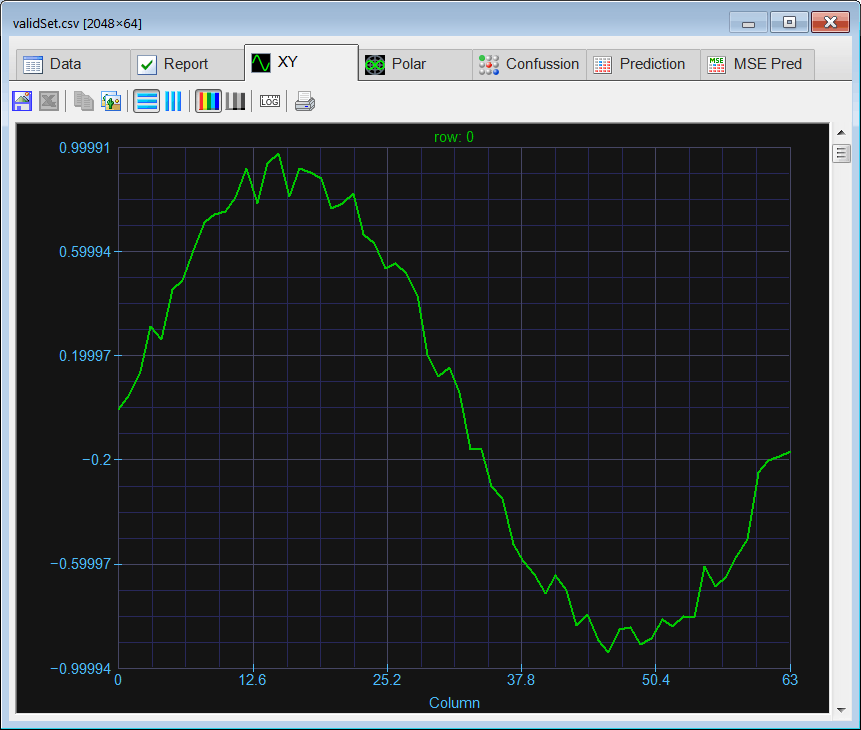Problem 3 Edit the Train.lab file to design and train an ANN for the sine wave. Use one hidden layer and 14 neurons in this layer. Train the ANN using simulated annealing and the conjugate gradient method. Because of the number of outputs, it is not possible to use the method of Levenberg Marquardt.

 Solution 3 After editing the file,Run click the button to execute the code. If you do not have any errors, the ANN will be trained when the code execution stops. Double click the network in the variable list to simulate the network, and then select the trainSet.csv file for input, and the trainSet.csv file for target. You will be able to review the network behavior in real time by moving the scrollbar at the right.

 SineWave\Train.lab int numInputs = 64; int i; //_________________________ Network Setup LayerNet net; net.Create(numInputs, 7, 0, numInputs); for(i = 0; i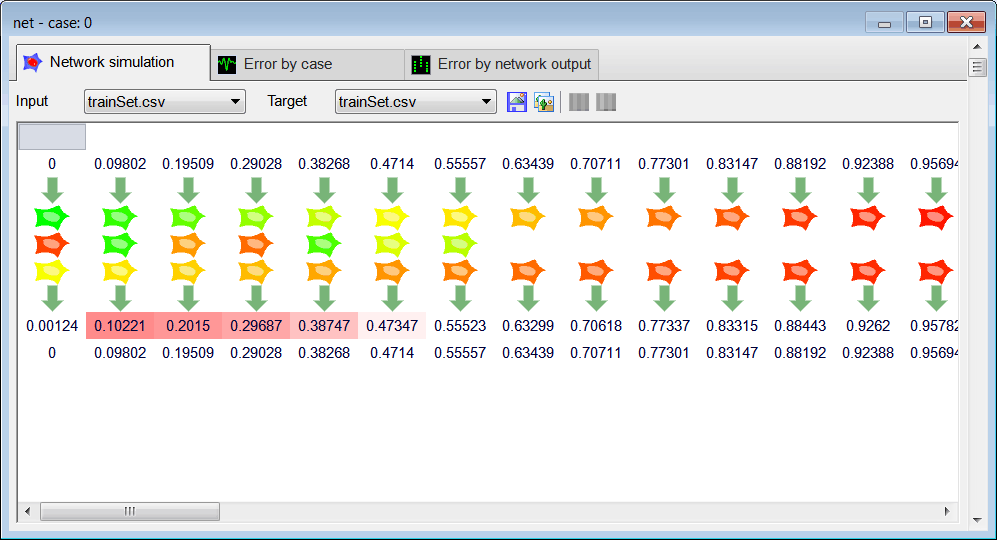Problem 4 Edit the CheckTraining.lab file to check the training: (a) Compute the mean squared error for the ANN using the training set. (b) Plot the error for each training case. (c) What conclusions can be obtained by looking at the error graph? (d) Save the plot as a vector image (checkTraining.pdf and checkTraining.emf)

 Solution 4 (a) After editing the file,Run click the button to execute the code. If you do not have any errors, the mse will be displayed in the variable list. Click the variable called output to review the network behavior. If you network was trained properly, you should be able to see that the output of the network is a perfect sine wave for all of training cases (use the scroll bar at the right to verify the output for other training cases). Do not forget to change the view to XY Plot to plot the output data.

 SineWave\CheckTraining.lab //_________________________________________ Load the training set Matrix trainSet; trainSet.Load(); //_________________________________________ Load the ANN LayerNet net; net.Load(); //_________________________________________ Run Matrix output = net.Run(trainSet); double mse = ComputeMse(output, trainSet); //_________________________________________ Relative Error Vector error = ComputeRelError(output, trainSet); XyChart checkTraining; checkTraining.SetCaption("case", false, "Relative Error", false); checkTraining.AddGraphY(error, "Relative Error", 2, 1, 0, 255, 0); checkTraining.SetLogScale(false, true); checkTraining.SetLimits(0, trainSet.GetRowCount(), 1.0e-5, 1.0); checkTraining.SetColorMode(2); checkTraining.SaveAndShow(); checkTraining.SavePDF(0.0); checkTraining.SaveEMF();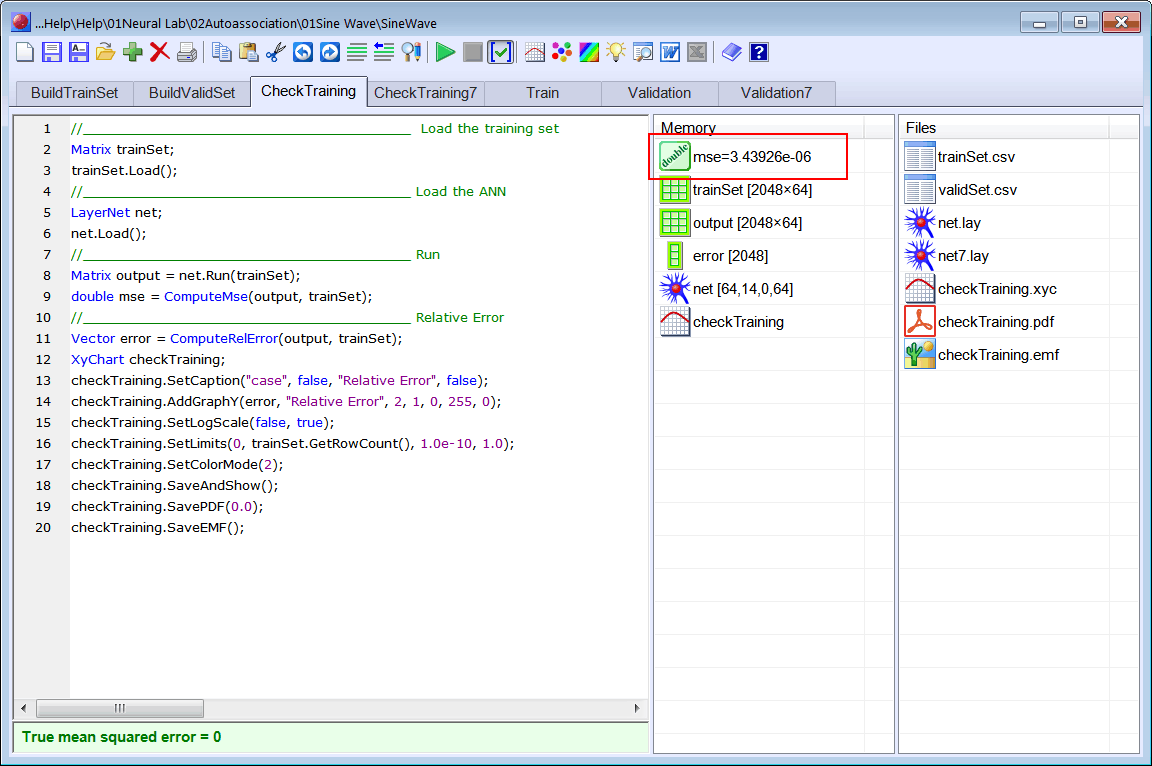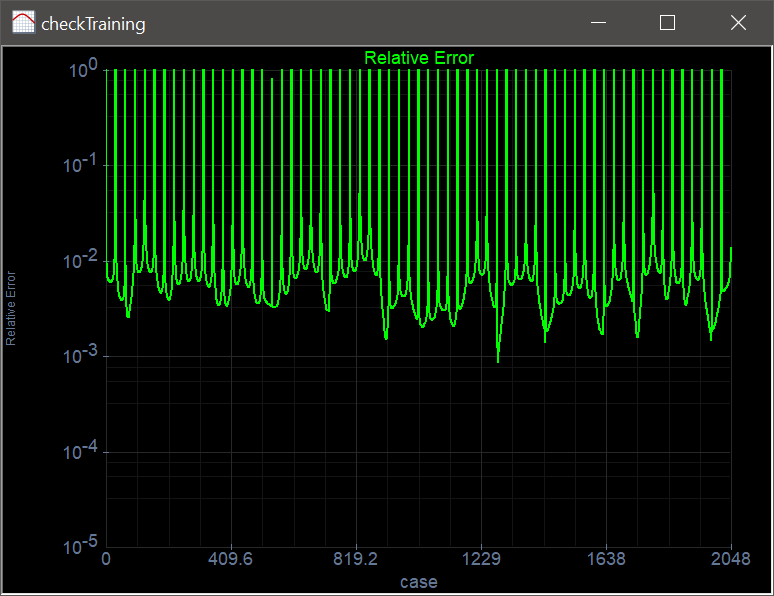Problem 5 Edit the Validation.lab file to perform the validation of the ANN. (a) Compute the mean squared error for the ANN using the validation set. (b) Plot the error for each validation case. (c) What conclusions can be obtained by looking at the error graph? (d) Save the plot as a vector image (validation.pdf and validation.emf)

 Solution 5 (a) After editing the file,Run click the button to execute the code. If you do not have any errors, the mse will be displayed in the variable list. As the value of the mse for both the training set and the validation set is NOT similar, there is something wrong in the way the ANN has been used for auto-association. Click the variable called output to review the network behavior. As the network was NOT properly designed and trained, the output of the network may not be a sine wave.

 SineWave\Validation.lab //_________________________________________ Load the validation set Matrix validSet; validSet.Load(); //_________________________________________ Load the ANN LayerNet net; net.Load(); //_________________________________________ Run Matrix output = net.Run(validSet); //_________________________________________ Compute the mean squared error double mse = ComputeMse(output, validSet); //_________________________________________ Relative Error Vector error = ComputeRelError(output, validSet); XyChart validation; validation.SetCaption("case", false, "Relative Error", false); validation.AddGraphY(error, "Relative Error", 2, 1, 0, 255, 0); validation.SetLogScale(false, true); validation.SetLimits(0, validSet.GetRowCount(), 1.0e-5, 1.0); validation.SetColorMode(2); validation.SaveAndShow(); validation.SavePDF(0.0); validation.SaveEMF();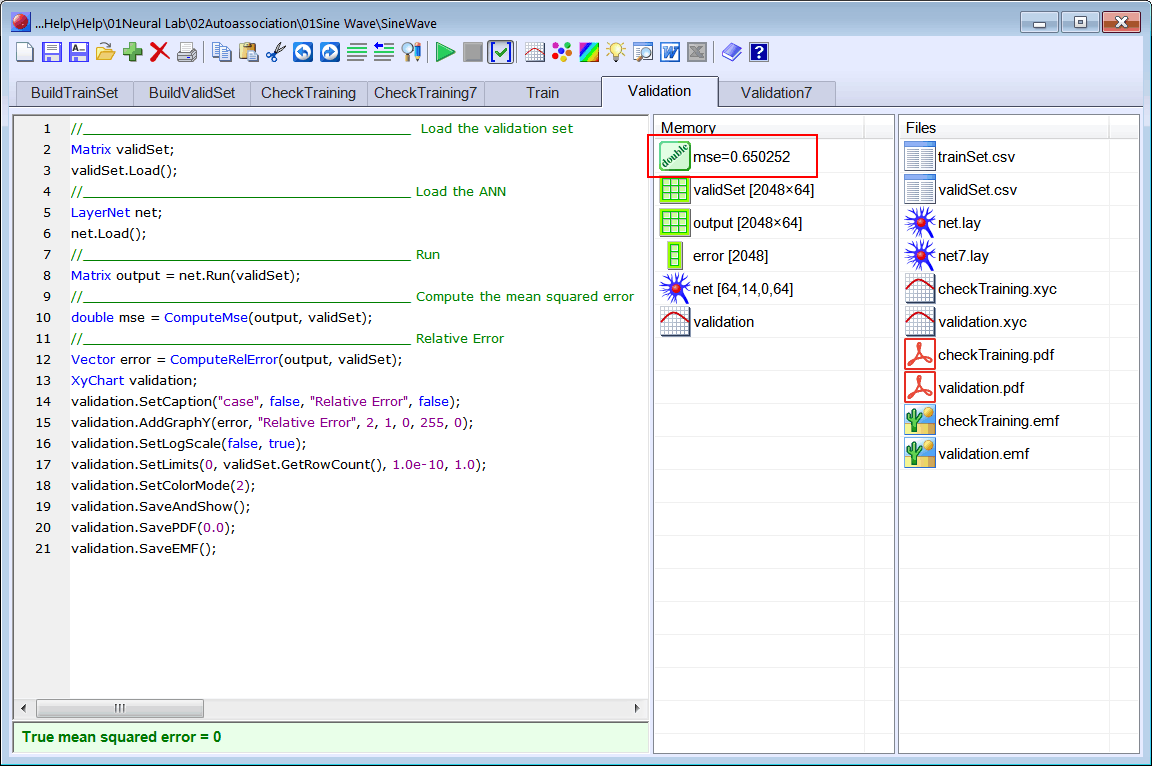Problem 6 Change the number of neurons in the hidden layer to 7 and train again the ANN.

 Problem 7 Check the training for the network with 7 neurons in the hidden layer: (a) Compute the mean squared error for the ANN using the training set. (b) Plot the error for each training case.

 Problem 8 Perform the validation for the network with 7 neurons in the hidden layer: (a) Compute the mean squared error for the ANN using the validation set. (b) Plot the error for each validation case.

 Problem 9 Try to figure out what is wrong with the design and training of the ANN. What can be done to improve the performance of the ANN?

 Problem 10 Generate a report in Microsoft Word. Write some conclusions in the report focusing on the problems that were faced during the simulation and how these problems were or could be solved.